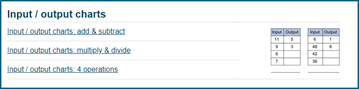# Math Drill Worksheets

Many of you have asked for worksheets to practice drilling the basic math facts as well as multi-digit arithmetic. Rather than include these in our grade-by-grade math sections, we’ve created an entirely new section dedicated to just math drills.

In this section you’ll find five sections. The first four are divided into two sub-sections each. The first is dedicated to the basic math facts, and the second section is dedicated to multi-digit addition, subtraction, multiplication and division. These sections are so large we’ll introduce each in future blog posts.

## Mixed four operations worksheets

The fifth section is a little different. We’ve created three sub-sections to deliver mixed math facts, mixed four operations, and order of operations worksheets separately. Let’s take deeper dive into the mixed math facts practice.

## Mixed math facts practice

The worksheets in this area provide mixed practice of the basic math facts:

Mixed addition and subtraction facts, including adding and subtracting within 10 and then all the math basic math facts on the vertical and the horizontal.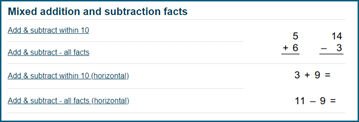Similarly, for mixed multiplication and division facts, we’ve created horizontal and vertical worksheets, ranging from small numbers to larger ones.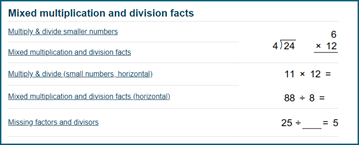The next section contains all four operations math facts worksheets.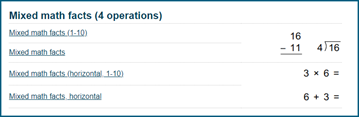The fact family worksheets help students to learn the relation between addition/subtraction math facts, and multiplication/division math facts.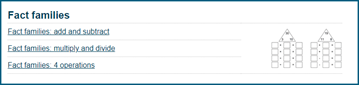Finally, a set of input/output charts help students in learning about the addition, subtraction, multiplication and division rules used to produce the sums. Like the fact family worksheets, these worksheets help students to understand the relation between the operations.